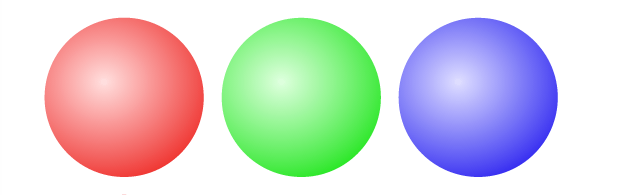# How Many Positions on a Rubik’s Cube?

## A fun lesson in permutations

The Rubik’s Cube is a challenging puzzle to solve, largely because there are so many positions. Wouldn’t it be fun to know exactly how many positions there are? Wouldn’t it be even more fun to know how to calculate that number for yourself?

# Back To The Basics

Imagine we have three marbles and each marble is a different color. One is red, another is green, and the third is blue. If we were to place the marbles in a row on the table in front of us, how would we arrange them?The ordering that we’ve selected is just one possible position of many. We might have chosen to put green first instead of red. Each of these positions is a permutation of our marbles. If we were to rearrange the marbles, then we would find another permutation.

“A permutation of a set of objects is an arrangement of those objects into a particular order." - wikipedia

In order to...